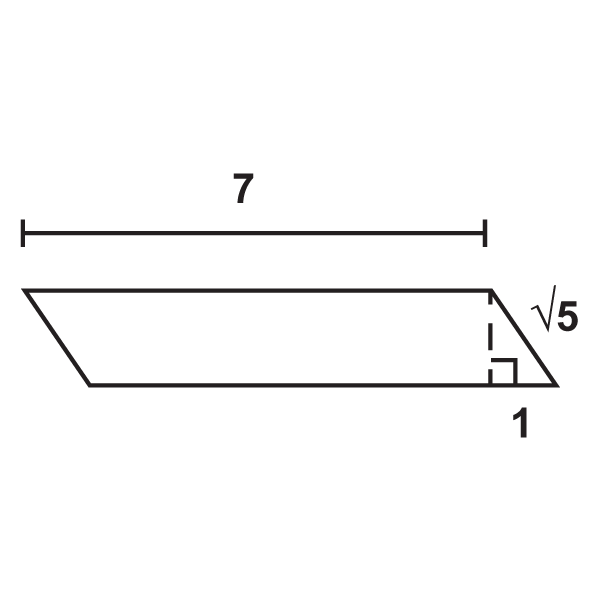# 5.10: Area of a Parallelogram

•• Contributed by CK12
• CK12

Use $$A=bh$$ to find area.

A parallelogram is a quadrilateral whose opposite sides are parallel.Figure $$\PageIndex{1}$$

To find the area of a parallelogram, make it into a rectangle.Figure $$\PageIndex{2}$$

From this, we see that the area of a parallelogram is the same as the area of a rectangle. The area of a parallelogram is $$A=bh$$. The height of a parallelogram is always perpendicular to the base. This means that the sides are not the height.Figure $$\PageIndex{3}$$

What if you were given a parallelogram and the size of its base and height? How could you find the amount of space the parallelogram takes up?

Example $$\PageIndex{1}$$

Find the area of the parallelogram.Figure $$\PageIndex{4}$$

Solution

Area is $$15(6)=90\text{ un}^2$$.

Example $$\PageIndex{2}$$

Find the area of the parallelogram with a base of 10 m and a height of 12 m.

Solution

Area is 10(12)=120\text{ m}^2\).

Example $$\PageIndex{3}$$

Find the area of the parallelogram.Figure $$\PageIndex{5}$$

Solution

$$A=15\cdot 8=120 \text{ in}^2$$

Example $$\PageIndex{4}$$

If the area of a parallelogram is $$56 \text{ units }^2$$ and the base is 4 units, what is the height?

Solution

Solve for the height in $$A=bh$$.

$$56\text{ units }=4h$$

$$14 \text{ units }=h$$

Example $$\PageIndex{5}$$

If the height of a parallelogram is 12 m and the area is $$60 m^2$$, how wide is the base?

Solution

Solve for the base in $$A=bh$$.

$$60 \text{ units } =12b$$

$$5 \text{ units } =b$$

## Review

1. Find the area of a parallelogram with height of 20 m and base of 18 m.
2. Find the area of a parallelogram with height of 12 m and base of 15 m.
3. Find the area of a parallelogram with height of 40 m and base of 33 m.
4. Find the area of a parallelogram with height of 32 m and base of 21 m.
5. Find the area of a parallelogram with height of 25 m and base of 10 m.

Find the area of the parallelogram.

1.Figure $$\PageIndex{6}$$
2.Figure $$\PageIndex{7}$$
3.Figure $$\PageIndex{8}$$
4.Figure $$\PageIndex{9}$$
5.Figure $$\PageIndex{10}$$
6.Figure $$\PageIndex{11}$$
7.Figure $$\PageIndex{12}$$
8. If the area of a parallelogram is $$42\text{ units }^2$$ and the base is $$6\text{ units }$$, what is the height?
9. If the area of a parallelogram is $$48\text{ units }^2$$ and the height is $$6\text{ units }$$, what is the base?
10. If the base of a parallelogram is 9 units and the area is $$108\text{ units }^2$$, what is the height?
11. If the height of a parallelogram is 11 units and the area is $$27.5\text{ units }^2$$, what is the base?

## Vocabulary

Term Definition
area The amount of space inside a figure. Area is measured in square units.
perimeter The distance around a shape. The perimeter of any figure must have a unit of measurement attached to it. If no specific units are given (feet, inches, centimeters, etc), write units.
Parallelogram A parallelogram is a quadrilateral with two pairs of parallel sides.
Area of a Parallelogram The area of a parallelogram is equal to the base multiplied by the height: $$A = bh$$. The height of a parallelogram is always perpendicular to the base (the sides are not the height).

Interactive Element

Video: Area of a Parallelogram (Whole Numbers)

Activities: Area of a Parallelogram Discussion Questions

Study Aids: Triangles and Quadrilaterals Study Guide

Practice: Area of a Parallelogram

Real World: Perimeter